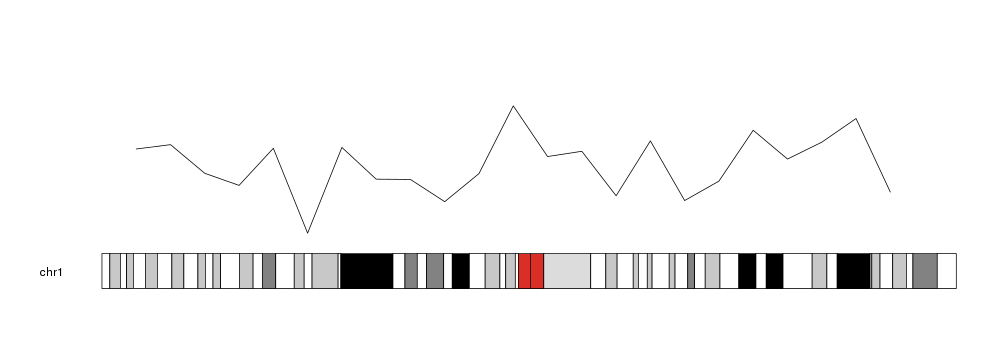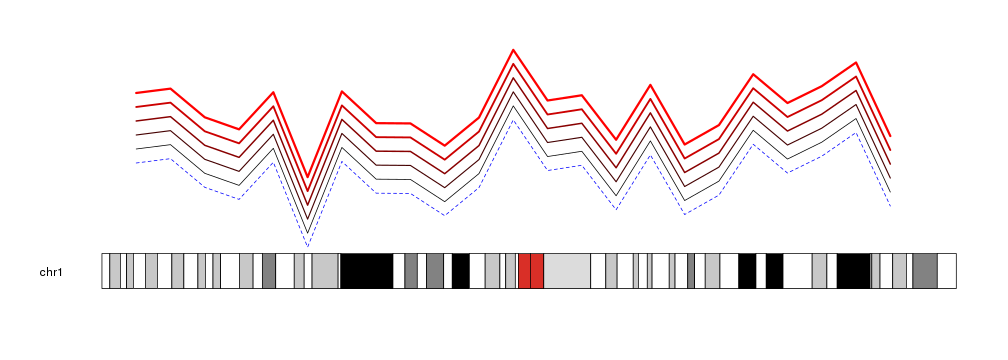Plotting Lines

To plot lines in a karyoplot we need to use the kpLines function. Given a character vector chr and two integer vectors x and y it will plot a single line joining the points specified.

library(karyoploteR)

x <- 1:23*10e6
y <- rnorm(23, mean=0.5, sd=0.25)

kp <- plotKaryotype(chromosomes="chr1")
kpLines(kp, chr="chr1", x=x, y=y)Lines are cutomizable with the same graphical parameters as in the R base graphics lines function: lwd, lty, col

kp <- plotKaryotype(chromosomes="chr1")
kpLines(kp, chr="chr1", x=x, y=y)
kpLines(kp, chr="chr1", x=x, y=y+0.1, col="#440000", lwd=1.5)
kpLines(kp, chr="chr1", x=x, y=y+0.2, col="#880000", lwd=2)
kpLines(kp, chr="chr1", x=x, y=y+0.3, col="#CC0000", lwd=2.5)
kpLines(kp, chr="chr1", x=x, y=y+0.4, col="#FF0000", lwd=3)
kpLines(kp, chr="chr1", x=x, y=y-0.1, col="blue", lty=2)